# 【人臉辨識】使用5 Facial Landmarks進行臉孔校正

face alignment 的功能主要是將傾斜的人臉旋轉至端正的角度，用以提高後續進行人臉識別時的精準度。在進行 alignment 前，需要先取得 facial landmarks 才能進行校準，目前最常用的 facial landmark model 則是 Dlib 所提供的 5 點及 68 點模型，一般多是使用 68 點 landmarks 來進行校正。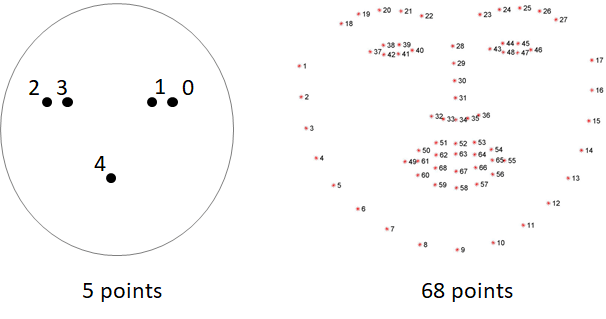Dlib 提供的 5 點及 68 點 facial landmark model（圖片來源：曾成訓提供）

### Dlib Facial Landmarks 的效率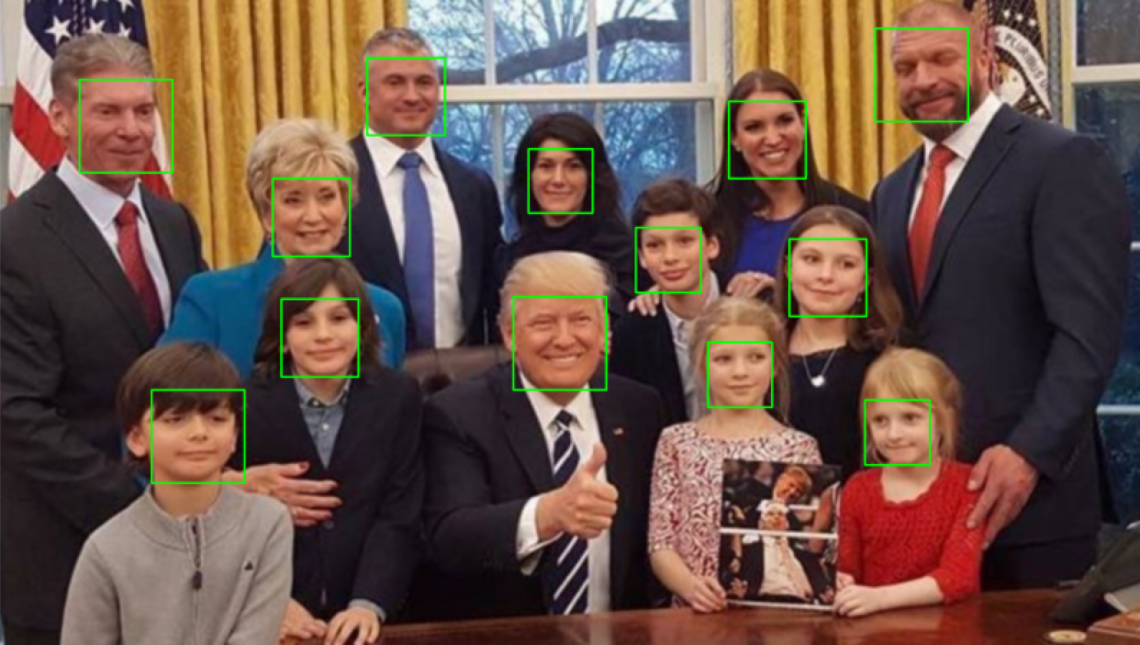（圖片來源：曾成訓提供）（圖片來源：曾成訓提供）（圖片來源：曾成訓提供）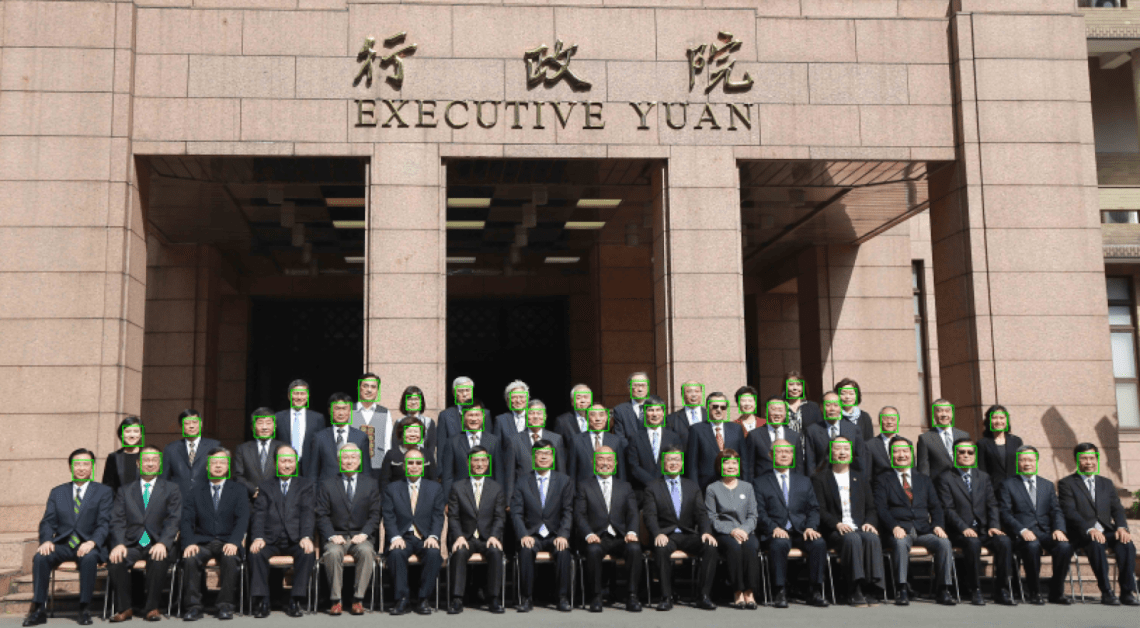（圖片來源：曾成訓提供）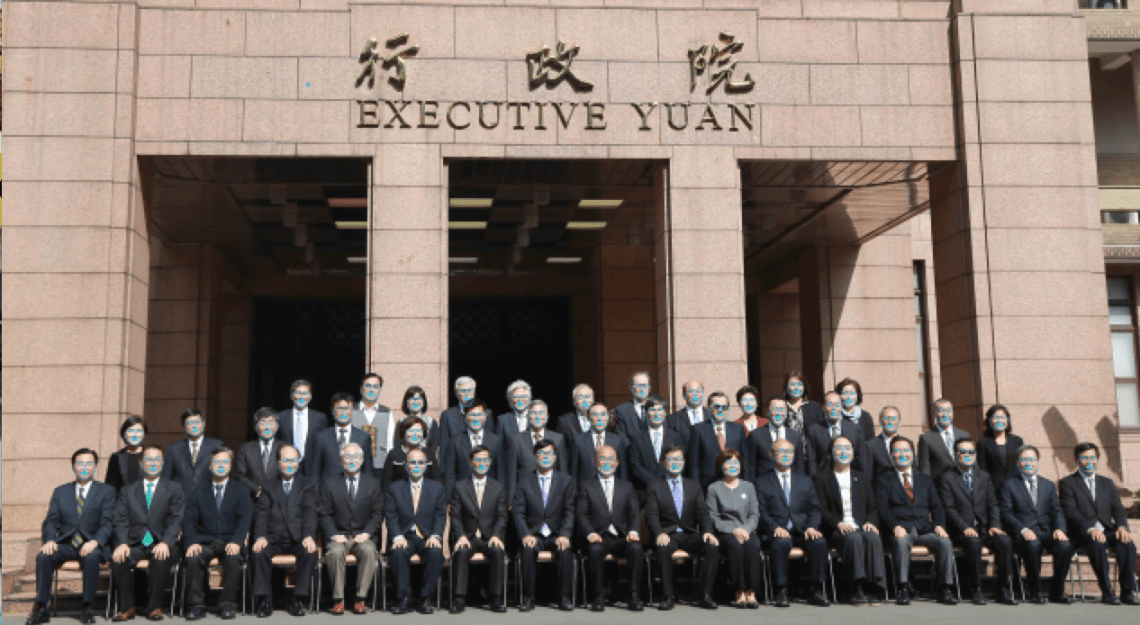（圖片來源：曾成訓提供）（圖片來源：曾成訓提供）

### 取得 Facial Landmarks

import dlib 後，只需要宣告臉孔偵測及 landmarks 偵測物件後就可以開始使用了，唯一要注意的是，dlib 所回傳的 bounding box 及 points 是專屬的格式，使用時需注意：

1. 臉孔偵測：Dlib 回傳某個臉孔的 bbox 物件為 dets，因此該臉孔的（x,y,w,h）為（d.left(), d.top(), d.right, d.bottom()）。
2. Landmarks 偵測：Dlib 回傳某個臉孔的 landmarks 物件為 shape，如果想取得每個點的（x,y），可透過如下的方式：landmarks = np.matrix（[[p.x, p.y] for p in shape.parts()]）

```import dlib
import cv2

#Dlib facial landmarks model的path
predictor_path = "models/shape_predictor_5_face_landmarks.dat"
#待處理的相片
face_path = "faces/IMG_2995.jpg"

#於landmarks上畫圓，標識特徴點
def renderFace(im, landmarks, color=(0, 255, 0), radius=3):
for p in landmarks.parts():
cv2.circle(im, (p.x, p.y), radius, color, -1)

#detector為臉孔偵測，predictor為landmarks偵測
detector = dlib.get_frontal_face_detector()
predictor = dlib.shape_predictor(predictor_path)

#讀入相片
#偵測臉孔
dets = detector(img, 1)

#針對相片中的每張臉孔偵測五個landmarks
for k, d in enumerate(dets):
shape = predictor(img, d)
renderFace(img, shape)

cv2.imshow("face-rendered", img)
cv2.waitKey(0)```

### 進行對齊 Alignment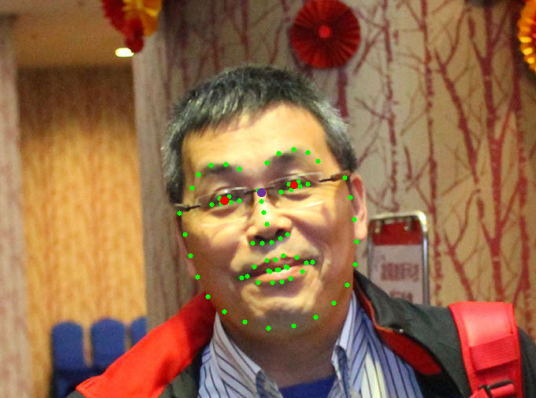（圖片來源：曾成訓提供）

1. 取得左右兩眼的中心點

leftEyeCenter = leftEyePts.mean(axis=0).astype(“int”)

rightEyeCenter = rightEyePts.mean(axis=0).astype(“int”)

2. 依據此兩點形成的直線，計算與水平線的角度

dY = rightEyeCenter – leftEyeCenter

dX = rightEyeCenter – leftEyeCenter

angle = np.degrees(np.arctan2(dY, dX)) – 180

3. 依雙眼間距計算旋轉後的縮放比例（依使用者所指定對齊後的臉孔大小）

desiredRightEyeX = 1.0 – self.desiredLeftEye

dist = np.sqrt((dX ** 2) + (dY ** 2))

desiredDist = (desiredRightEyeX – self.desiredLeftEye)

desiredDist *= self.desiredFaceWidth

scale = desiredDist / dist

4. 取得雙眼的中間點，此點亦作為臉部旋轉的中心點

eyesCenter = ((leftEyeCenter + rightEyeCenter) // 2,

(leftEyeCenter + rightEyeCenter) // 2)

5. 計算稍後要使用於 cv2.warpAffine 的旋轉矩陣 M

M = cv2.getRotationMatrix2D(eyesCenter, angle, scale)

tX = self.desiredFaceWidth * 0.5

tY = self.desiredFaceHeight * self.desiredLeftEye

M[0, 2] += (tX – eyesCenter)

M[1, 2] += (tY – eyesCenter)

6. 套用旋轉矩陣 M，完成臉部的校正對齊

(w, h) = (self.desiredFaceWidth, self.desiredFaceHeight)

output = cv2.warpAffine(image, M, (w, h), flags=cv2.INTER_CUBIC)

Adrian Rosebrock 有將此 face alignment 的程式碼放在 imutils 的模組中，因此只要 import 進來後，輸入幾行指令便能套用此功能。

```import cv2
import imutils
import dlib
import numpy as np
from imutils.face_utils import FaceAligner
from imutils import face_utils

predictor_path = "models/shape_predictor_5_face_landmarks.dat"
face_path = "faces/IMG_2884.jpg"

detector = dlib.get_frontal_face_detector()
predictor = dlib.shape_predictor(predictor_path)
fa = FaceAligner(predictor, desiredFaceWidth=256)

image = imutils.resize(image, width=800)
gray = cv2.cvtColor(image, cv2.COLOR_BGR2GRAY)

cv2.imshow("Input", image)
rects = detector(gray, 2)

for rect in rects:
(x, y, w, h) = face_utils.rect_to_bb(rect)
faceOrig = imutils.resize(image[y:y + h, x:x + w], width=256)
faceAligned = fa.align(image, gray, rect)

cv2.imshow("Original", faceOrig)
cv2.imshow("Aligned", faceAligned)
cv2.waitKey(0)```

### 改用 5 points landmarks 進行 aligment

1. 取得雙眼左右兩點的 landmarks
2. 依 landmarks 計算雙眼的中間點
3. 計算兩個中間點與水平線的角度
4. 重新取得較大的人臉區域，並依第三步所得到的角度旋轉
5. 針對旋轉後的區域重新取得人臉，此即為 aligned 的人臉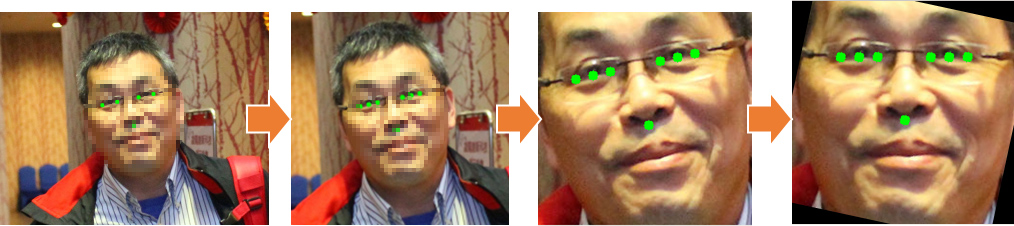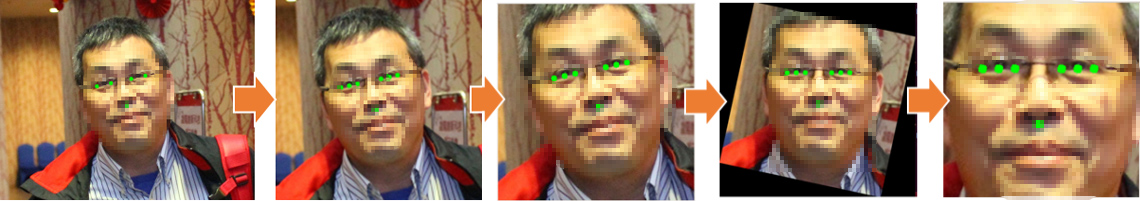### 相關程式說明

• Dlib 臉部偵測

```detector = dlib.get_frontal_face_detector()
def detect_face(face_area):
bbox = []
dets = detector(face_area, 1)
for k, d in enumerate(dets):
print("Detection {}: Left: {} Top: {} Right: {} Bottom: {}".format(
k, d.left(), d.top(), d.right(), d.bottom()))
bbox.append((d.left(), d.top(), d.right()-d.left(), d.bottom()-d.top()))

return bbox
```
• 繪製特徵點

```def renderFace(im, landmarks, color=(0, 255, 0), radius=5):
for p in landmarks:
cv2.circle(im, (p, p), radius, color, -1)```
• 計算兩點角度

```def angle_2points(p1, p2):
r_angle = math.atan2(p1 - p2, p1 - p2)
rotate_angle = r_angle * 180 / math.pi

return rotate_angle
```
• 擴張 bounding box 區域（臉部區域）
```def area_expand(bbox, ratio):
ew = int(bbox * ratio)
eh = int(bbox * ratio)
nx = int(bbox - ((ew - bbox) / 2))
ny = int(bbox - ((eh - bbox) / 2))
if(nx<0):
nx = 0
if(ny<0):
ny = 0

return (nx,ny,ew,eh)```

### 小結

（本文經作者同意轉載自 CH.TSENG 部落格、原文連結；責任編輯：賴佩萱）Author: 曾 成訓